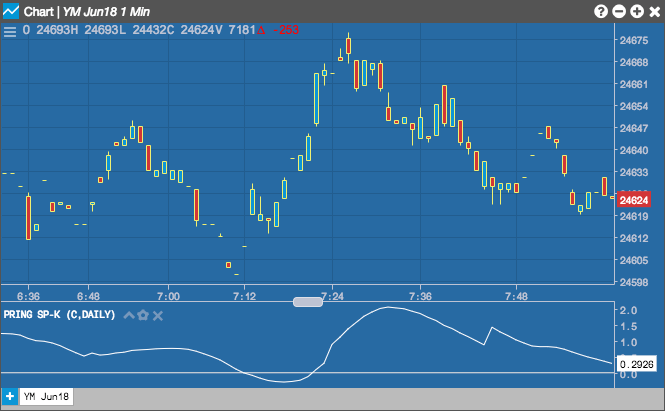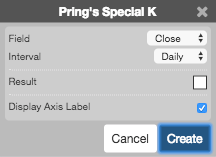Charts

# Pring's Special K

The Pring's Special K indicator combines short-term, intermediate and long-term velocity into one complete series, thereby giving us true summed cyclicality. It has two functions: to identify primary trend reversals at a relatively early stage and to use that information for timing short-term pro-trend price moves.## Configuration Options• Field: Price or combination of prices to use as the base for average calculations. Possible values include:
• Open
• High
• Low
• Close
• HL/2 $$\left ( \frac{High + Low}{2} \right )$$
• HLC/3 $$\left ( \frac{High + Low + Close}{3} \right )$$
• HLCC/4 $$\left ( \frac{High + Low + Close + Close}{4} \right )$$
• OHLC/4 $$\left ( \frac{Open + High + Low + Close}{4} \right )$$